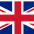## Thank you for subscribing!

It's great to feel loved.en

Nov 05, 2020 4 min read

Some times when using the very popular Google sheet, you have to subtract in Google sheet some values and it seems a bit difficult to do, well here are some tips on how to make your experience on Google spreadsheet more glamorous. Generally, subtraction in Google sheet can be done in two forms using;

## Using the Subtraction Formula

For Google sheet subtracts, you just have to choose a cell where you want to place the result of your calculations. In the bar for the formula, input an equal sign followed by the digits you intend to subtract. This has to be done quite carefully in other to avoid unwanted errors in your calculations.

For the Google sheet subtraction formula, at the point, you intend to place the formula result, pick a cell. Put down an equal sign followed by numbers that you are looking to subtract in the formulas bar. When convenient, exchange cell references into the formula. You also have to separate terms with the hyphen symbol, which will stand to serve as the minus sign.## Subtraction with Minus Function

We can all agree that Excel has its own unique function but when it comes to Google spreadsheets, the minus function is quite operational here with respect to excel, there is just one important factor to note, for spreadsheets, only two values can be worked upon at a time. This is a limitation, therefore the minus function can only be used when you don't have so much to calculate.

It cannot also work for complicated terms. Using an example:

If in cell A4 there is sixty and in cell B4 there is 20 when the minus function is inputted, cell C4 will become 40 and that is for '=minus(A4, B4)' if this is taken the other way, a value of -40 is gotten. This means that even a negative number can be gotten on this spreadsheet. Subtraction Google sheet is possible using the minus function## How Do I Calculate Differences in Google Sheet

When trying to get or calculate the differences in the Google sheet, you can find the difference between two distinct numbers by bringing in a formula in a blank cell.

Let's take an example, assuming A5 and B5 are distinct values, A1 could easily minus B2 and you bring in an equal sign in front of A1 and that will do the job. There is no specific order in the arrangements. You can also go both ways to get a different answer.

## How Do I Subtract in Spread Sheet

Subtraction in Google sheets can be done quite easily as stated before, two methods are known and if more than one value is to be subtracted, you might want to use the "subtraction formula" because the "minus function" can only work for two variables.

Spreadsheets have been known to accommodate quite a large range of numerical calculations, it's why it is mostly employed in a complex situation, and in such situations, the subtraction formula is always employed.## Conclusion

For the subtract formula in Google sheet, which was spoken about, since it can work with varying distinct numerical values, instead of using the numeric values you can make reference to the cells in which such values are placed, it can help in varying degrees to help reduce congestion since it can resolve functions as it does for math.

It is also relevant to note that there are no limits to the numbers, the functions, and also terms that it can accommodate. This has made Google sheet the go-to guy when it comes to complex calculations in financial reports and the likes.Written by Mike

Former data analyst and the head of Whatagraph blog team. A loving owner of two huskies, too.

Google docs are not only easy to use and share but also have many useful features. One of these valuable features is google docs find and replace, which allows you to replace any words or phrases you want to change.